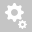##Recently viewed tickets# Compression-only Multi-linear Springs

Question:

How do we simulate compression-only multi-linear springs for modeling p-y,T-z, and Q-z curves of a multi-layered soil system. We are trying to perform soil-structure interaction analyses of a pile bent with 54-inch cylinder piles embedded into this soil system.

As you will note from the model, we are using multi-linear point spring supports with the following backbone curves - Symmetric for T-z (friction), Unsymmetric for Q-z (bearing with Dz:-ve) and p-y (lateral). How do we ensure that the lateral springs (p-y) are deactivated when subjected to lateral tension force (since soil cannot take tension) under the test load.

Thanks.

This can be done in two ways.

As highlighted in the image below, the first option of directly selecting compression only spring and the stiffness could be enters.

the second option is using the multi-linear spring itself with unsymmetrical properties.
i.e compression is positive so for positive input in x stiffness in y should be provides.
So for tension , x is in negative and if the spring has to compression only then y- ordinate corresponding to tension would be zero.

Please note that ax<bx<cx ... and similarly ay <=by<=cy<=...----------------------------------------------------------------------------------------------------------------------------------------------------------------------------------------------

Question 2:

As suggested by you, Option 1 cannot be used since soil curves are non-linear.

Option 2 is tested using a cantilever beam with its free end supported by a point spring with unsymmetric backbone curve. The signs of Dz (associated with the point spring) are made +ve for an expected compression-only behavior and -ve for tension-only behavior. Based on your pointer, reference to the Midas user manual and our understanding of the verbiage, the reaction force at the point spring should essentially be zero when Dz is defined as -ve (i.e., when the point spring is set as tension-only. However, as you will note the reaction forces in the beam remain the same regardless of whether we set Dz as +ve or -ve. Please clarify.

I have considered a different example to indicate the difference.
Considered is a continuous beam , with same uniform load applied

1) In -ve Z direction. The multilinear spring is considered at the middle support. Now under this UDL , tension should develop at this spring , but the stiffness being zero for tension there is no reaction and it simply behaves as a beam just supported at both ends.2) Now if I apply the UDL in +ve Z direction , the middle support would have compression and hence the springs come into play , generating reaction and behaves as a continuous beamFind attached the model for reference.

Hope this helps. DO let us know if any further clarification is required.
Creation date: 10/27/2017 7:24 AM ()      Updated: 10/27/2017 5:00 PM ()